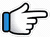# Dil Ibadat Kar Raha Hai – Keyboard Notes## Dil Ibadat Kar Raha Hai Keyboard NotesFor Dil Ibadat Kar Raha Hai Notes as Western or Indian Notations, Click –

Western –
Indian –

## Keyboard Notes for Dil Ibadat Kar Raha Hai – Indian Notation (srg mpdn)

Music:
rgmpd pd pd
s*r*  p
mp  mg

d      m*g*~ m*   s*     g*r*   s*

N~ NN          s*N       d

dpm       g         r~       d      dd
Tujhko_ main_ kar   loon_hasil

dN       N       pd       p
Lagi     hai    yahi     dhun.
Or
dN        N       dp      d

Notes for Full Line:
d   m*g*~ m*   s*   g*r*   s*,   N~ NN   s*N   d

dpm   g   r~   d    dd,    dN   N   pd    p
Tujhko_ main_ kar   loon_hasil,  Lagi  hai yahi  dhun.

d~ m*g*m*    s*g*     r*    s*
Zindgi_ ki      shakh    se    loon

N~          NN        s*      N         d
Kuchh    hasin    pal    main    chun

dpm       g         r          d      dd
Tujhko_ main_ kar   loon_  hasil

dN       N      pd        p
Lagi     hai    yahi    dhun.

Notes for Full Line:
d~ m*g*m*   s*g*    r*   s*,     N~     NN    s*    N     d
Zindgi_ ki shakh se loon, Kuchh hasin pal main chun

dpm   g   r   d    dd,   dN   N   pd  p
Tujhko_ main_ kar loon_ hasil, Lagi hai yahi dhun.

Music:
rgmpd pd pd
s*r*  p
mp  mg

d      m*g*~ m*   s*     g*r*   s*

N~ NN           s*N     d

dpm       g         r~      d      dd
Tujhko_ main_ kar   loon_hasil

dN       N       pd       p
Lagi     hai    yahi    dhun.

d~ m*g*m*   s*g*       r*    s*
Zindgi_ ki      shakh    se    loon

N~          NN        s*      N         d
Kuchh    hasin    pal    main    chun

dpm       g         r          d      dd
Tujhko_ main_ kar   loon_  hasil

dN      N      pd       p
Lagi    hai    yahi   dhun.

r*     g*     m*~ m*~
Jo    bhi    jitne

m*     p*m*g*r*
pal     jeeyu

r*g*     m*~m*~    m*     p*m*g*r*
Unhe    tere          sang_  jeeyu

p*    g*      g*     g*     r*r* g*r*s*
Jo    bhi    kal    ho    ab    mera

s*s*     NN      s*        Nd
Use      tere    sang    jeeyu

r*     g*     m*~ m*~
Jo    bhi    saanse

m*        p*m*g*r*
main    bharoo

r*g*     m*~ m*~     m*   p*m*g*r*
Unhe    tere            sang _ bharoo

p*g*       g*   g*    r*r* g*r*s*
Chahe    jo    ho    ra~sta

s*s*    NN     s*        Nd
Use     tere   sang    chalu

d       m*g*~ m*   s*     g*r*    s*,     N~ NN           s*N     d

dpm       g         r~       d      dd,         dN       N       pd      p
Tujhko_ main_ kar   loon_hasil,     Lagi     hai    yahi    dhun.

d~ m*g*m*    s*g*    r*   s*,          N~         NN        s*     N        d
Zindgi_ ki    shakh    se    loon,    Kuchh    hasin    pal    main    chun

dpm       g         r       d    dd,         dN        N      pd       p
Tujhko_ main_ kar loon_ hasil,   Lagi     hai    yahi    dhun.

g*r*           r*     r*   r*r* r*g*r*s*
Mujhko    de    tu    mit    jaane

s*r* g*     r*        r*    r*r* r*g*r*s*
Ab    khud_se    dil    mil    jaane

s*r*     g*     r*      r*g*m*   g*r*s*Ns*N
Kyu    hai    yeh    itna         faasla~~

g*r*r*      r*      r*       r* r*g*r*s*
Lamhe    yeh    phir   naa    aane
Or
g*r*r*   r*    r*      r* r*r*s*

s*r*g*      r*     r*       r*     r*g*r*s*
Inko         tu    naa    de      jaane

s*r*    g*         r*     r*g*m*   g*    r*s*Ns*N
Tu      mujh    pe    khudko    de    loota~~.

r*g*     m*m*     m*    p*m*g*r*
Tujhe    tujhse    tod    loo

r*g*    m*m*      m*     p*m*g*r*
Kahi    khudse    jod    loo

p*p*      p*    g*    r*     r*        g*r*s*
Mere    jism   o    jaan_main   aa

s*s*    NN              s*      Nd
Teri     khusboo    odh    loo

r*     g*      m*~ m*~,        m*   p*m*g*r*,   r*g*
Jo    bhi     saanse,            main    bharoo,

m*~ m*~     m*       p*m*g*r*,
Unhe           tere      sang _ bharoo

p*g*      g*    g*    r*r* g*r*s*,    s*s*   NN   s*        Nd
Chahe    jo    ho    ra~sta,            Use     tere     sang    chalu

d      m*g*~ m*   s*     g*r*   s*,     N~ NN      s*N     d

dpm       g         r~      d      dd,       dN     N      pd      p
Tujhko_ main_ kar   loon_hasil,     Lagi     hai    yahi    dhun.

Will be Updated:
Bahoon main de bas jaane
sine main de chhup jaane
tujh bin main jaaunga kaha

Tujhse he mujhe ko paane
ek jinpe haq ho bas mera

tere khwabo main jagoo
mujhe doondhe jab koi
teri aankho main milu

r*    g*     m*~ m*~,    m*   p*m*g*r*,
Jo    bhi     saanse,     main    bharoo,

r*g*      m*~ m*~  m*   p*m*g*r*,
Unhe    tere          sang _ bharoo

p*g*     g*   g*    r*r* g*r*s*,   s*s*   NN   s*        Nd
Chahe    jo    ho    ra~sta,               Use     tere     sang    chalu

d      m*g*~ m*   s*     g*r*   s*,     N~ NN      s*N     d

dpm       g         r~      d      dd,       dN     N      pd      p
Tujhko_ main_ kar   loon_hasil,     Lagi     hai    yahi    dhun.

d~ m*g*m*    s*g*     r*   s*,       N~        NN     s*     N        d
Zindgi_ ki    shakh    se    loon,    Kuchh    hasin    pal    main    chun

dpm       g         r         d     dd,     dN     N      pd      p
Tujhko_ main_ kar  loon_ hasil,   Lagi     hai    yahi    dhun.